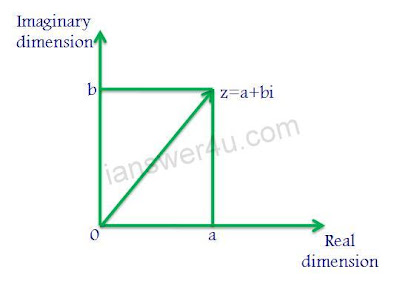# What Are Complex Numbers? Examples and Solving techniques

### Define: Complex numbers

Complex numbers are the numbers which have both real and imaginary parts. The imaginary part of the complex number can’t be represented on number line. They are of the form a+ bi where a and b are real numbers while i is an imaginary part (i = √-1), “a” represents the real part while "bi" represents the imaginary part.
Complex were introduced to find the solution of equation
X2 + 1 = 0
Here x=√-1, that is represented as i.

Two complex numbers x+iy and a+ib are said to be equal if their real parts are equal and their imaginary parts are also equal, i.e. x=a and y=b.

### Representation of Complex NumbersComplex numbers on Argand Plane

In the Argand plane, the vertical axis is called the imaginary axis while the horizontal axis is called the real axis. The real numbers are complex numbers with imaginary part equal to zero.
Complex Numbers are used in engineering and other scientific calculations.